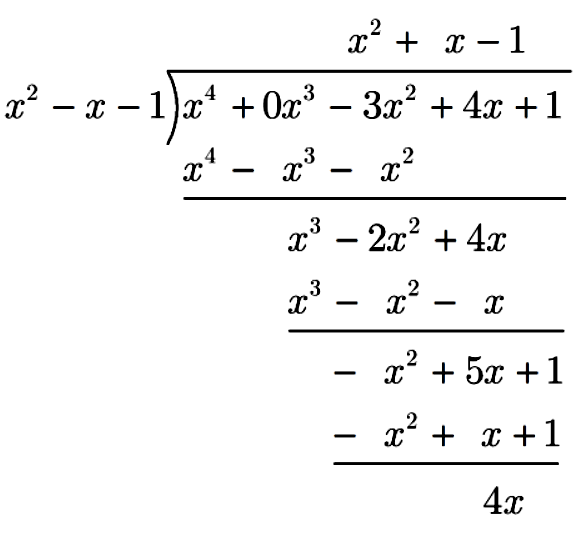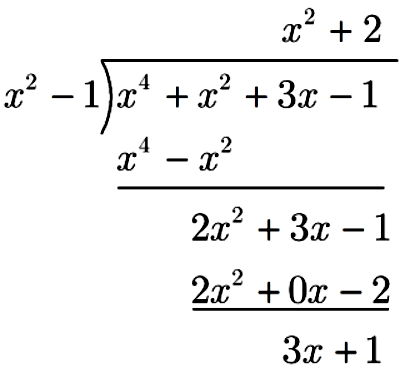## Featured Posts

### MCQ Questions : The Remainder Theorem and The Factor Theorem

မှန်သော အဖြေကို ရွေးပေးရန် ဖြစ်ပါသည်။

 1. The degree of a polynomial is the _____ A. largest coefficient of $x$ B. smallest coefficient of $x$ C. highest power of $x$ D. lowest power of $x$ Explanation ကိန်းရှင် $x$ ပါဝင်သော polynomial တစ်ခုတွင် $x$ ၏ အကြီးဆုံးထပ်ညွှန်းကို ၎င်း polynomial ၏ degree (သို့) order ဟု ခေါ်သည်။ When $9 x^{2}-6 x+2$ is divided by $x-3$, the remainder will be A. $60$ B. $\dfrac{15}{2}$ C. $\dfrac{19}{5}$ D. $65$ Explanation Let $f(x)=9 x^{2}-6 x+2$. When $f(x)$ is divided by $x-3$, The remainder = $f(3)=9 (3)^{2}-6 (3)+2=65$ If a polynomial $f(x)$ is divided by a linear divisor $(x-a)$, then the remainder is A. $f(-a)$ B. $f(a)$ C. $a$ D. $f(0)$ Explanation Remainder Theorem ကို မေးထားခြင်း ဖြစ်ပါသည်။ For what value of $k, x+4$ is a factor of the polynomial $x^{2}-x-(2 k-2) ?$ A. $7$ B. $-9$ C. $11$ D. $-5$ Explanation $x+4$ is a factor of the polynomial $x^{2}-x-(2 k-2)$. By factor theorem, $(-4)^{2}-(-4)-(2 k-2)=0$ $16+4-2(k-1)=0$ $2(k-1)=20$ $k=11$ If the two roots of the equation $x^{3}-x^{2}-5 x+5=0$ are $\sqrt{5}$ and $-\sqrt{5}$, then the third root is A. $1$ B. $-1$ C. $2$ D. $-2$ Explanation The two roots of the equation $x^{3}-x^{2}-5 x+5=0$ are $\sqrt{5}$ and $-\sqrt{5}$. Let the third root be $k$. $\therefore \quad x^{3}-x^{2}-5 x+5=\left(x-\sqrt{5}\right)\left(x+\sqrt{5}\right)\left(x-k\right)$. $\therefore\quad \sqrt{5}\times \left(-\sqrt{5} \right)\times (-k)=5$ $\quad\quad 5k=5$ $\quad\quad k=1$ What should be subtracted to the polynomial $x^{2}-16 x+30$, so that $x-15$ is the factor of the resulting polynomial? A. $30$ B. $14$ C. $15$ D. $16$ Explanation Let $f(x) = x^{2}-16 x+30$. When $f(x)$ is divided by $x-15$, The remainder = $f(15) = (15)^{2}-16 (15)+30 = 15(15 - 16 + 2) = 15$ Hence, $15$ should be subtracted. The quadratic polynomial $a x^{2}+b x+c=0$ where $c=\dfrac{b^{2}}{4 a}$ has _____real roots. A. $1$ B. $2$ C. $0$ D. None of these. Explanation $a x^{2}+b x+c=0$ where $c=\dfrac{b^{2}}{4 a}$ $\therefore\quad 4ac = b^2$ $\therefore\quad b^2-4ac = 0$ $\therefore\quad a x^{2}+b x+c=0$ has only one root. The remainder when $x^{4}-3 x^{2}+4 x+1$ is divided by $x^{2}-x-1$ is A. $x$ B. $2x$ C. $3x$ D. $4x$ ExplanationIf the sum and the product of the roots of $x^{2}+b x+c=0$ are $3$ and $2$ , then A. $b=-3, c=2$ B. $b=3, c=2$ C. $b=-3, c=-2$ D. $b=3, c=-2$ Explanation The sum and the product of the roots of $x^{2}+b x+c=0$ are $3$ and $2$. Let the roots be $p$ and $q$. $\therefore\quad p+q = 3, \ pq=2$ $\therefore\quad x^{2}+b x+c=(x-p)(x-q)$ $\therefore\quad x^{2}+b x+c=x^{2}-(p+q) x+pq$ $\therefore\quad x^{2}+b x+c=x^{2}-3x+2$ $\therefore\quad b = -3, \ c = 2$ Given that $f(x)=x^{4}+x^{2}+3 x-1$ and $g(x)=x^{2}-1$. If $f(x)=q(x) g(x)+r(x)$, which of the following is true? $\mathbf{I.}\ q(x)=x^2+2 \quad$ $\mathbf{II.}\ r(x)=3 x+1 \quad$ $\mathbf{III.}\ g(x)$ is a factor of $f(x)$ A. I only B. II only C. I and II only D. I and III only Explanation$f(x)=x^{4}+x^{2}+3 x-1$ and $g(x)=x^{2}-1$ $\quad\quad f(x)=q(x) g(x)+r(x)$ (given) $\therefore\quad f(x)=(x^2+2)(x^2-1)+3x+1$ $\therefore\quad q(x)=x^2+2, \ r(x) = 3x+1$ If $x-3$ is a factor of $x^{3}-(2+k) x^{2}+7 k$, then $k=$ A. $4$ B. $4.5$ C. $5$ D. $5.5$ Explanation $x-3$ is a factor of $x^{3}-(2+k) x^{2}+7 k$. $\therefore\quad 3^{3}-(2+k) (3)^{2}+7 k=0$ $\therefore\quad 27-9\left(2+k\right)+7k=0$ $\therefore\quad k=\dfrac{9}{2} = 4.5$ If $f(x)=6 x^{3}+13 x^{2}+p x+q$ is exactly divisible $2 x^{2}+7 x-4$, then A. $3 x-4$ is a factor of $f(x)$ B. $3 x+4$ is a factor of $f(x)$ C. $4 x-3$ is a factor of $f(x)$ D. $4 x+3$ is a factor of $f(x)$ Explanation $f(x)=6 x^{3}+13 x^{2}+p x+q$ is exactly divisible $2 x^{2}+7 x-4$ $2 x^{2}+7 x-4=(2x-1)(x+4)$ $\therefore\quad 6 \left(\dfrac{1}{2}\right)^{3}+13 \left(\dfrac{1}{2}\right)^{2}+p \left(\dfrac{1}{2}\right)+q=0 \ldots(1)$ $\quad\quad 6 \left(-4\right)^{3}+13 \left(-4\right)^{2}+p \left(-4\right)+q=0\ldots(2)$ $\therefore\quad p=-40,\ q=16$ $\therefore\quad f(x)=6 x^{3}+13 x^{2}-40x+16$ $\therefore\quad f \left(\dfrac{4}{3}\right)=6 \left(\dfrac{4}{3}\right)^{3}+13 \left(\dfrac{4}{3}\right)^{2}-40\left(\dfrac{4}{3}\right)+16$ $\hspace{2.7cm} =\dfrac{128}{9}+\dfrac{208}{9}-\dfrac{160}{3}+16$ $\hspace{2.7cm} =0$ $\therefore\quad 3 x-4$ is a factor of $f(x)$. If $x^{2}+2 x-3$ is a factor of $f(x)=x^{4}+2 x^{3}-7 x^{2}+a x+b$, then A. $a=8$ and $b=12$ B. $a=-8$ and $b=12$ C. $a=8$ and $b=10$ D. $a=-8$ and $b=10$ Explanation $x^{2}+2 x-3$ is a factor of $f(x)=x^{4}+2 x^{3}-7 x^{2}+a x+b$ Let $f(x) = g(x)(x^{2}+2 x-3)$ $\therefore x^{4}+2 x^{3}-7 x^{2}+a x+b = g(x)(x+3)(x-1)$ When $x=-3,\ (-3)^{4}+2 (-3)^{3}-7 (-3)^{2}+a (-3)+b = 0$ When $x=1,\ (1)^{4}+2 (1)^{3}-7 (1)^{2}+a (1)+b = 0$ $\therefore\quad 3a - b =-36 \ \ldots(1)$ $\quad\quad a + b = 4 \ \ \quad\ldots(2)$ $\therefore\quad a= -8, b=12$ If $x^{2}+a x+b$ is divided by $x+c$, then remainder is A. $c^{2}-a c-b$ B. $-c^{2}-a c+b$ C. $c^{2}+a c+b$ D. $c^{2}-a c+b$ Explanation $x^{2}+a x+b$ is divided by $x+c$, then the remainder $= (-c)^{2}+a (-c) +b = c^2-ac+b$ If $x^{3}+9 x+5$ is divided by $x$, then the remainder is A. $9$ B. $5$ C. $-9$ D. $0$ Explanation $x^{3}+9 x+5$ is divided by $x$, then the remainder $= (0)^{3}+9 (0)+5 = 5$ If $(x-1)$ is a factor of $a x-a$, then the value of $a$ is A. $1$ B. $2$ C. $3$ D. any real number Explanation Let $f(x) = ax - a$ $f(1) = a - a = 0$ $\therefore\quad x-1$ is a factor of $f(x)$ for all value of $a\in \mathbb{R}$. What is the remainder when $f(x)=x^{3}+3 x^{2}+k x+k$ is divided by $p x$ ? A. $f(p)$ B. $f\left(\dfrac{1}{p}\right)$ C. $k$ D. $f(k)$ Explanation When $f(x)=x^{3}+3 x^{2}+k x+k$ is divided by $p x$, The remainder = $f(0) = k$ If $x^{2}-3 x+2$ is a factor of the polynomial $f(x)$, then $f(2)=$ A. $0$ B. $1$ C. $2$ D. None of these Explanation $x^{2}-3 x+2$ is a factor of the polynomial $f(x)$. $x^{2}-3 x+2 = (x-1)(x-2)$ $\therefore\quad (x-1)$ and (x-2) are factors $f(x)$. $\therefore\quad f(2)=0$ Given that $f(x)=q(x)\left(x^{2}-4\right)+3$. What is the remainder when $f(x)$ is divided by $x-2$ ? A. $1$ B. $3$ C. $2$ D. $0$ Explanation $f(x)=q(x)\left(x^{2}-4\right)+3$. When $f(x)$ is divided by $x-2$, The remainder $f(2)=q(2)\left(2^{2}-4\right)+3 =3$ If $n$ is a positive integer, what is the remainder when $5 x^{2 n+1}+10 x^{2 n}-3 x^{2 n-1}+5$ is divided by $x+1$. A. $13$ B. $-1$ C. $5$ D. $10$ Explanation Let $f(x)=5 x^{2 n+1}+10 x^{2 n}-3 x^{2 n-1}+5$ where $n$ is a positive integer. When $f(x)$ is divided $x + 1$, The remainder $= f(-1)$. $\hspace{2.5cm} =5 (-1)^{2 n+1}+10 (-1)^{2 n}-3 (-1)^{2 n-1}+5$ $\hspace{2.5cm} =5 (-1)^{\text{odd integer}}+10 (-1)^{\text{even integer}}-3 (-1)^{\text{odd integer}}+5$ where $n\in \mathbb{J^+}$ $\hspace{2.5cm} =5 (-1)+10 (1)-3 (-1) + 5$ $\hspace{2.5cm} =13$ Your Score:
Previous
Next Post »

## သင်ရိုးသစ် လေ့ကျင့်ခန်း နှင့် အဖြေများ

Chapter 1 - Coordinate Geometry
Chapter 2 - Exponents and Radicals
Chapter 3 - Logarithms
Chapter 4 - Functions